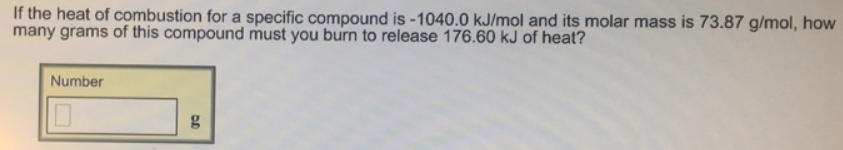Chemistry Calorimetry Solution: If the heat of combustion for a specific compound ...

# Solution: If the heat of combustion for a specific compound is -1040.0 kJ/mol and its molar mass is 73.87 g/mol, how many grams of this compound must you burn to release 176.60 kJ of heat?

Problem

If the heat of combustion for a specific compound is -1040.0 kJ/mol and its molar mass is 73.87 g/mol, how many grams of this compound must you burn to release 176.60 kJ of heat?View Complete Written Solution